Technical Report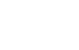Vibration and Sensors I

1. What is Vibration?

Vibration is classified into three types:

·Linear Vibration
·Bending Vibration
·Torsional Vibration

In order to treat such vibrations quantitatively, we use the following three parameters: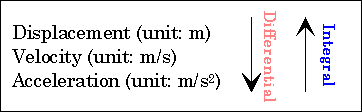Each parameter can be transformed into another by differential or integral. If velocity is constant over the frequency, displacement and acceleration versus frequency are as described in the graph below .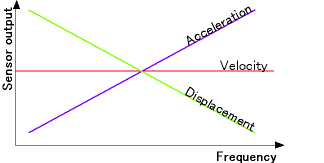This graph shows displacement is most sensitive at a low frequency, but that sensitivity of acceleration exceeds that of the others at a high frequency.This means that for sensitive measurement, displacement should be used at low frequencies; and acceleration should be used at high frequencies. For applications such as plant diagnosis, displacement and velocity are used at frequencies under several hundred hertz and acceleration is used at higher frequencies.

2. Choice of Vibration Sensor

To choose the best vibration sensor for your needs, consider the following points:

* What should be measured?

Displacement
Velocity
Acceleration

* Object Size

There are two types of sensors: contact type and non-contact type. When you use a contact-type sensor, you should consider mass effect (will be described later). In addition, regardless of a sensor type, you should consider measurement size of the sensor (S) and object size (S'). If S'/S is not larger than 1, exact measurement will be impossible.

* Amplitude of Vibration and Frequency Range

You should estimate the amplitude and frequency range of the object because miscalculation could damage the sensor.

* Environment

You should check the ambient temperature and humidity as well as temperature of the object and whether the environment is free from dust, oil, or water. Some measurement methods can be affected by these factors.

Those considerations will help you choose the best sensor for your needs. For convenience, our product line is shown below.

Products correspondence table

Methods Capacitance Eddy Current Laser Piezoelectric Electromagneic
Trigonometrical Doppler Charge output Built-in Amp. Single sensor Double sensor
Model VE series VS series LD series LV series NP-2000 series NP-3000 series MP/PD series
Non-contact possible possible possible possible
Vibration Type Linear possible possible possible possible possible possible
Torsional possible possible
Physical Quality Displacement possible possible possible possible
Velocity possible possible
Acceleration possible possible
Frequency Low good good good possible possible possible possible possible
High possible possible possible good good
Ultra-high good
Amplitude Large possible good good
Small good possible possible
Environment High-temp. possible
Dust-proof possible

Notes

·Contact sensors and Non-contact sensors

Contact sensor

·Advantages : Relatively cheep, easy to use

·Caution : Mass effect

Mass effect refers to the sensor mass ability to affect an object's natural frequency.
Because natural frequency depends on mass, an object to which sensor is attached will have a lower natural frequency than that of the object itself. If a sensor mass is too large, the sensor will decrease the object natural frequency, thus resulting in measurement error. If the object's mass, sensor mass, and the system's natural frequency be M, m, and fe, respectively, and the natural frequency will decrease by delta fe according to the equation shown in the figure below. It is recommended that the sensor mass be under one-fiftieth of the object's mass. When m is one-fiftieth of M, the frequency deviation, delta fe/fe, amounts to 0.01. Note that M is NOT the mass of the whole object but the mass of the part to which the sensor will be attached. The part may be unexpectedly light.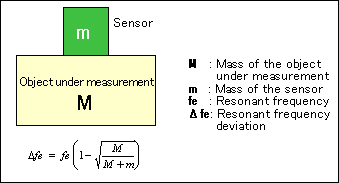Non-contact sensor

·Advantage : No effect on vibration
Non-contact sensors can be effectively used to measure objects, such as a rotator, which a contact sensor cannot measure.

·Caution : Sensors should be isolated from vibrations.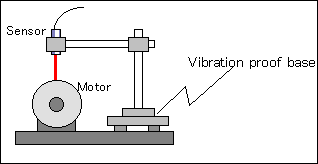3. Principles and Features of Vibration Sensors

(1)The principles and features of the sensors listed in the above table are as follows:

Principle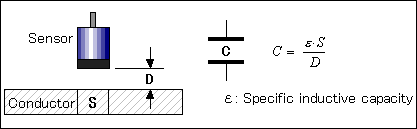This sensor uses the capacitance between the sensor and the object to measure the gap (displacement). Therefore the object is limited to a conductor. Capacitance C is a function of the opposed conductor area S and the gap D. If the sensor and the object are parallel flat plates, we can use the above equation. The gap D is inverse proportional to the capacitance C if the area S is considered to be constant. Thus we can obtain the gap D by measuring the capacitance C.

* Non-contact measurement
* No calibration required for all conductors
* High accuracy and high stability
* Even an extremely small gap can be measured.
* Measurement possible at DC

Cautions

* Conductivity needed
* Measurement easily influenced by oil, water and other substances on surface of the object
* Relatively large measurement area

(2) Eddy Current Sensor (VS series) - Displacement Sensor -

Principle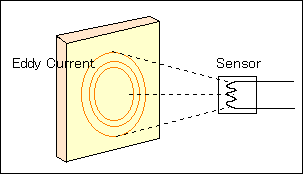Since this sensor uses eddy current, the material of the object is limited to metal. The LC resonant circuit composed of the sensor coil inductance L and the transducer capacitance C is made resonant by a crystal oscillator. If a metal object is close to the high-frequency coil, the alternating magnetic field in the coil generates eddy current in the metal. Since this eddy current depends on the magnetic force at the metal surface, or the distance between the coil and the object, the inductance L will change with the eddy current, which varies the terminal voltage of the resonant circuit as a function of the distance. Detecting this signal will give the gap from the sensor to the object.

* Non-contact measurement
* For all metals
* Immune to water and oil on the object surface
* Operation possible at high temperature
* Measurement possible at DC

Cautions

* Relatively large measurement area
* Mutual interference of two or more sensors adjacent to each other

(3) Laser (LD series) - Displacement Sensor -

Principle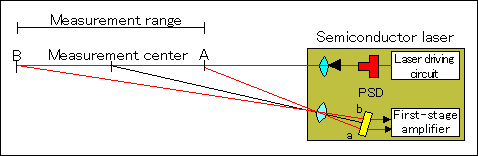This is a displacement sensor using optical trigonometry. Using a laser as a light source ensures high accuracy and high stability. The structure of this sensor is shown by the figure above. When the laser arrives at the object surface, part of the diffusely reflected light is focused on a position sensing detector (PSD). The object positions at A (near) and B (distant) will make a focused spot at a and b, respectively, on the PSD. We can measure the object displacement by detecting the spot that varies with the displacement.

* Non-contact measurement
* Small measurement area
* Able to measure large amplitude
* Measurement possible at DC

Cautions

* Has difficulty in measuring small amplitude
* Not able to measure at high frequency (up to 2kHz)

(4) Piezoelectric Sensor (Charge output/Built-in Amplifier) (NP-2000/3000 series)

Principle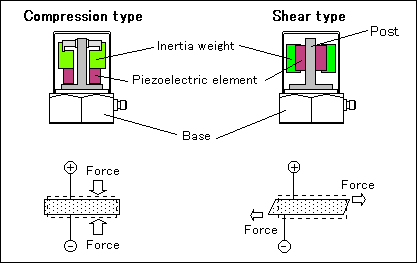External force generates electric changes on the surface of such materials as single crystal and barium titanate. This is called piezoelectric effect, and such materials are called piezoelectric materials (piezoelectric devices). A piezoelectric acceleration pickup uses a piezoelectric device as a spring for a seismic system and a mechano-electric transducer. This sensor outputs an electric signal proportional to vibration acceleration. The piezoelectric acceleration pickup is classified by the force applying direction into two major types: compression type and shear type. The figures above show their structures; the compression type has a piezoelectric device located between a sensor base and a weight. The shear type has a piezoelectric device fixed between a post perpendicular to a base and a weight. The compression type was popular in the past, but the shear type has pervaded recently because of immunity to base distortion and rapid changes in temperature. Most of our piezoelectric acceleration pickups are of the shear type.

* Fairly easy to operate
* Inexpensive set up
* High stability, with few secular changes

Cautions

* Mass effect and contact resonance should be considered because this is a contact sensor
* Impossible to measure a motion with constant acceleration

Dependence of frequency characteristics on sensor fixing ways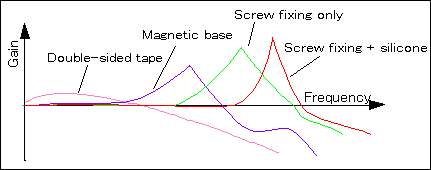How a sensor is fixed will affect the sensor frequency characteristics; see the above figure. You should choose the set up that best meets the frequency range needed for your measurement.

We will discuss a laser Doppler vibration sensor in our next report, as well as a torsional vibration sensor.